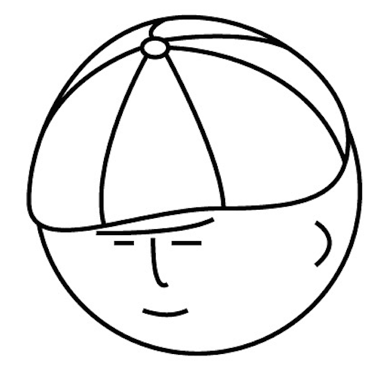# マツシタのお勉強メモ

#### マツシタのお勉強

##### welcome to my engineering blog
###### Hacker Rank# Find the next node (in-oder traversal) of a given node in a binary search tree

Problem Write an algorithm to find the next node (i.e., in-oder successor) of a given node in a binary search tree. You may assume that each node has a link to its parent. Solution This problem can be solved by thinking the two patterns. F…

# Check if a binary tree is a binary search tree

Problem Implement a function to check if a binary tree is a binary search tree Solution There are some solutions to solve this problem. I’ll introduce the all solution which includes I came up with. First Solution is using a range (min, ma…